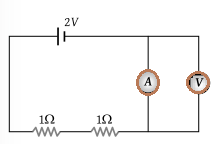# MCQ on Kirchhoffs Law for NEET

NEET  Physics is the scoring paper in the medical entrance examination. Here, you will discover the NEET Physics MCQ Questions for all Concepts as per the latest syllabus. Practice more on a regular basis with these NEET Physics objective questions on air pollution and improve your subject knowledge & problem-solving skills along with time management. NEET Physics Kirchhoffs Law Multiple Choice Questions make you feel confident in answering the question in the exam & increases your scores to high.

## MCQ on Kirchhoffs Law

1. Calculate the voltage across the 5ohm resistor(a) 12 V
(b) 4 V
(c) 10 V
(d) 0 V

2. Which basic law should be followed to analyse the circuit?
(a) Newton’s law
(c) Amperes law
(d) Kirchoff’s law

3. Calculate the value of V1 and V2(a) 4V, 6V
(b) 5V,6V
(c) 6V, 7V
(d) 7V, 8V

4. In Kirchhoff’s first law Σi = 0 at the junction is based on the conservation of
(a) Energy
(b) Charge
(c) Momentum
(d) Speed

5. In the circuit shown below what will be the reading in the voltmeter(a) 2 V
(b) 1 V
(c) 0.5 V
(d) Zero

6. What is the relation between currents in the figure below(a) i2 = i1 + i3 + i4 +i5
(b) i2 – i1 = i3 – i4 +i5
(c) i3 +i4 =i1+i2+i5
(d) I1+i5 =i2+i3+i4

Answer: (a) i2 = i1 + i3 + i4 +i5

7. The algebraic sum of voltages around any closed path in a network is equal to
(a) Infinity
(b) 1
(c) 0
(d) Negative polarity

8. Kirchhoff’s Current Law is based on
(a) The charge can be accumulated at the node
(b) Charge cannot be accumulated at the node
(c) Energy is stored at the node
(d) Depending on the circuit charge can be accumulated at the circuit

Answer: (b) Charge cannot be accumulated at the node

9. The terminal potential difference will be greater than its emf when it is
(a) In open circuit
(b) Being charged
(c) Being charged or discharged
(d) Being discharged